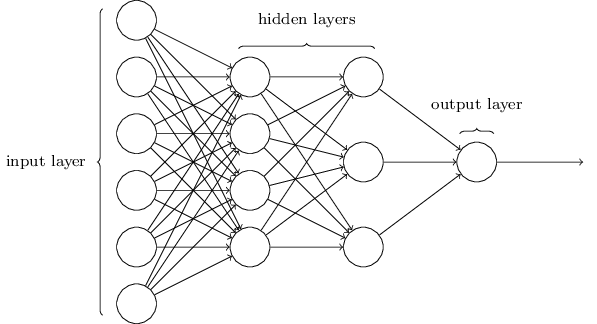# Feed Forward Networks

Feed forward neural networksFeed forward neural networks are the most common network architectures in predictive modeling, DynaML has an implementation of feed forward architectures that is trained using Backpropogation with momentum.

In a feed forward neural network with a single hidden layer the predicted target $y$ is expressed using the edge weights and node values in the following manner (this expression is easily extended for multi-layer nets).

\begin{equation} y = W_2 \sigma(W_1 \mathbf{x} + b_1) + b_2 \end{equation}

Where $W_1 , \ W_2$ are matrices representing edge weights for the hidden layer and output layer respectively and $\sigma(.)$ represents a monotonic activation function, the usual choices are sigmoid, tanh, linear or rectified linear functions.

The new neural network API extends the same top level traits as the old API, i.e. NeuralNet[Data, BaseGraph, Input, Output,Graph <: NeuralGraph[BaseGraph, Input, Output]] which itself extends the ParameterizedLearner[Data, Graph, Input, Output, Stream[(Input, Output)]] trait.

Tip

To learn more about ParameterizedLearner and other major model classes, refer to the model hierarchy specification.

In the case of NeuralNet, the parameters are a generic (unknown) type Graph which has to be an extension of NeuralGraph[BaseGraph, Input, Output]] trait.

Creating and training feed forward networks can be done by creating a back propagation instance and preparing the training data.

Tip

For a more in-depth picture of how the neural network API works refer to the neural stack page.

//Data is of some generic type
val data: DataType = _

//specify how this data can be
//converted to a sequence of input and output vectors.
val transform
: DataPipe[DataType, Seq[(DenseVector[Double], DenseVector[Double])]] = _

//Create the stack factory
//and back propagation instance
//Input, Hidden, Output
val breezeStackFactory = NeuralStackFactory(
Seq(5, 8, 3))(
Seq(VectorSigmoid, VectorTansig)
)

//Random variable which samples layer weights
val stackInitializer = GenericFFNeuralNet.getWeightInitializer(
Seq(5, 8, 3)
)

val opt_backprop =
new FFBackProp(breezeStackFactory)
.setNumIterations(2000)
.setRegParam(0.001)
.setStepSize(0.05)
.setMiniBatchFraction(0.8)
.momentum_(0.3)

val ff_neural_model = GenericFFNeuralNet(
opt_backprop, data,
transform, stackInitializer
)

//train the model
ff_neural_model.learn()